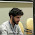## Wednesday, March 5, 2014

### DEFKTHON CTF 2014 - Find the FLAG! - Crypto 400 Write-up

HI!
We were given a lot of description this time :D and here they are:
Alice and Bob went a long way in crypto. They designed a super secure crypto system to encrypt their messages. We managed to steal the source and some other information. Find the FLAG!
File
Mirror
File
In the first file we had an example of the algorithm and the cipher we needed to decrypt and from the second file which is an archive we get 3 files 2 keys and the encryption script but script was obfuscated and names in the script were not readable to human, so lets start with de-obfuscating it - we did it by hand- and here is the a bit more readable script:

Basically it was reading four keys, and processing an asymmetric encryption on its first argument according to those keys.
The argument string is converted into a base256 number by those lines:
a, bb = argv, 0
for ccc in a: bb = (bb*256) + ord(ccc)
So what the encryption doing by mathematically is something like this:

$$T \equiv C^{A} \pmod{B}\\ P \equiv C^{S} \pmod{B}\\ Q \equiv M \cdot T^{S} \pmod{B}$$

where A,B,C and D are values in the keyfiles respectively and S is the random seed generated from D.

We know the values of B and C from the zip archive and P and Q from the message text that is containing an example and the cipher we need to find a way to get the value of M with those guys. Let's examine the $T^{S}$ actually it is equal to $(C^{A})^{S}$ and which is $P^{A}$ we just swapped the exponents. and last line became:

$$Q \equiv M \cdot P^{A}\pmod{B}$$

And besides those things we actually got one more very important data, the EXAMPLE! The example's P value and the P value of the cipher we need to decrypt are equal, also we know that A is equal for both of them so we can get the value of $P^{A}$ from the example -as we know Q and M for it. and then just multiply both sides of our equations with the inverse of the $P^{A}$ modulo B and then get the decrypted cipher lets do it now!

There's our python code for geting the flag:
http://www.codesend.com/view/7d97576bb77a0cc1ca33a717b6c8ed4d/

1.grateful
how find Pa value?
have Q=MP^A (mode B)
How will prove to be P^A=QM^(B-2) ???

2.P^A=QM^(B-2) (mod B)

3.Fermat's little theorem states that if p is a prime number:
$$a^{p-1} \equiv 1 \pmod{p}$$
So,
$$a^{p-2} \cdot a \equiv 1 \pmod{p}\\ a^{p-2} \equiv a^{-1} \pmod{p}$$

We can apply this theorem to our problem cause B is a prime, and in the:
$$Q \equiv M \cdot P^{A}\pmod{B}$$
equation if we multiply both sides with the inverse of $M$ then we'll get the $P^{A}$ we know in the example case all of those values. After getting $P^{A}$ we put its inverse in our flag cipher to get $M$.

4.In previous editions we have briefly described the products and services available at a few exchanges, to give you an idea of the different offerings. bitcoinvest.cc

5.BEST BITCOIN INVESTMENT SITE

Invest here Best Bitcoin investment site

6.This is such a great resource that you are providing and you give it away for free. I love seeing blog that understand the value of providing a quality resource for free. Sell Bitcoin

7.I can see that you are an expert at your field! I am launching a website soon, and your information will be very useful for me.. Thanks for all your help and wishing you all the success in your business. best cable company

8.Looks like the writer has put a lot of hard work into this.
9.